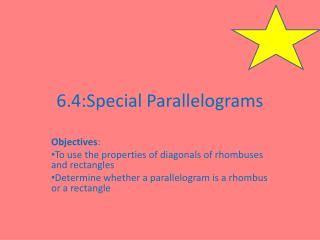DownloadDownload Presentation6.4:Special Parallelograms

# 6.4:Special Parallelograms

Download Presentation## 6.4:Special Parallelograms

- - - - - - - - - - - - - - - - - - - - - - - - - - - E N D - - - - - - - - - - - - - - - - - - - - - - - - - - -
##### Presentation Transcript

1. 6.4:Special Parallelograms Objectives: To use the properties of diagonals of rhombuses and rectangles Determine whether a parallelogram is a rhombus or a rectangle

2. Review of the Properties of a parallelogram: • 2 pairs of opposite parallel sides • 2 pairs of opposite congruent sides • Opposite angles are congruent • Consecutive angles are supplementary • Diagonals bisect each other Special Parallelograms: Rhombus, Rectangle, Square • These figures will inherit ALL the properties above, AND they will each add their own individual properties

3. Theorems For a Rhombus: • Each diagonal of a rhombus bisects 2 angles of the rhombus • The diagonals of a rhombus are perpendicular bisects & bisects & D C B A

4. Examples: Find the measures of the numbered angles in the rhombus. 1 12° 2 4 3

5. The figure below is a rhombus. Find TQ, TP, and SQ.

6. Theorem for Rectangle The diagonals of a rectangle are congruent. (remember, they also are bisected, so all 4 segments created by the intersection are congruent) AND E

7. EXAMPLE BD=5y-7 and AC = y + 5. Find the value of y and the length of BE. E

8. THEOREMS If one diagonal of a bisects 2 angles of the then the is a RHOMBUS. If the diagonals of a are perpendicular, then the is a RHOMBUS. If the diagonals of a are congruent, then the is aRECTANGLE.

9. SQUARE Remember, a square is a RECTANGLE and a RHOMBUS, so it inherits ALL the properties of a rectangle, rhombus and parallelogram.

10. Determine whether the quadrilateral can be a parallelogram. If not, write impossible. Explain your answer. • Each diagonal is 15 cm long, and one angle of the quadrilateral has a measure of 45°. • The diagonals are congruent, perpendicular, and they bisect each other.